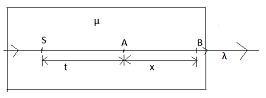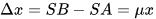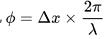Courses

# Test: Coherent And Incoherent Sources

## 10 Questions MCQ Test Physics For JEE | Test: Coherent And Incoherent Sources

Description
This mock test of Test: Coherent And Incoherent Sources for JEE helps you for every JEE entrance exam. This contains 10 Multiple Choice Questions for JEE Test: Coherent And Incoherent Sources (mcq) to study with solutions a complete question bank. The solved questions answers in this Test: Coherent And Incoherent Sources quiz give you a good mix of easy questions and tough questions. JEE students definitely take this Test: Coherent And Incoherent Sources exercise for a better result in the exam. You can find other Test: Coherent And Incoherent Sources extra questions, long questions & short questions for JEE on EduRev as well by searching above.
QUESTION: 1

### For sustained interference we need two sources which emit waves:​

Solution:

To obtain well-defined interference patterns, the intensity at points corresponding to destructive interference must be zero, while intensity at the point corresponding to constructive interference must be maximum. To accomplish this the necessary condition is that the two interference sources must be coherent, that is, they must keep a constant phase difference.

QUESTION: 2

### When the interference occurs with coherent sources, _______ of the superimposingwaves add like _______.

Solution:

The answer provided in the forum is wrong, The correct answer would be option A.
Solution:
When the interference occurs with non-coherent sources, intensities of the superimposing waves add like scalars, I = I1 + I2

QUESTION: 3

### Two sources of light are said to be coherent when both give out light waves of same:​

Solution:

In physics, two wave sources are perfectly coherent if they have a constant phase difference and the same frequency (amplitude may be different).
As c be the speed of light which is constant.
Using, c=νλ
Now same ν gives same λ. for the two light sources.
Example: y1​=A1​sinwt  and y2​=A2​sin(wt+ϕ) where ϕ is constant.

QUESTION: 4

Phase difference between two coherent sources should be:​

Solution:

Two sources are coherent if the waves they emit have the same wavelength and maintain a constant phase difference, ϕ. If the constant phase difference. ϕ, is zero, the two sources are said to be in phase.

QUESTION: 5

Coherent sources can be created by the division of:

Solution:

Division of wave front where wave front is divided into two or more parts with the help of mirrors, lenses and prisms. The common methods are : Young's double slit arrangement, Fresnel's biprism method, Lloyd’s mirror method etc.

Division of amplitude where amplitude of the incoming beam is divided into two or more parts by partial reflection or refraction. These divided parts travel different paths and reunited later to produce interference. E.g. Newton's rings, Michelson’s interferometer etc.

QUESTION: 6

In a Fresnel’s biprism experiment, the two coherent sources are obtained by:

Solution:

When a light travels from one medium to another then deviation in the path of light known as refraction. And in the prism surrounding medium is air and material medium is glass due to the change of medium refraction will occur in prism.

QUESTION: 7

A 60.0 kHz transmitter sends an EM wave to a receiver 21 km away. The signal also travels to the receiver by another path where it reflects from a helicopter. Assume that there is a 180o phase shift when the wave is reflected. This situation will give ________.​

Solution:

Given,
Frequency f=60kHz=60000Hz
Velocity of EM wane=v=3x108 m/s
Wavelength λ
We have v=f x λ
3x108 m/s= 60000 Hz x λ
λ=5000m
λ=5km
Destructive interference.

QUESTION: 8

Light of wavelength λ in vacuum enters a medium of refractive index μ. Two points in this medium, lying along the path of this light, are at a distance x apart. The phase difference between these points is

Solution:λ is the wavelength of light in air.

Let t be the distance between points S and A.

Optical path length travelled by light via SBOptical path length travelled by light via SA = μt
Path difference between points  A and BNow  phase differenceHence,QUESTION: 9

Which of the following is not essential for two sources of light in Young’s double slit experiment to produce a sustained interference?

Solution:

For sustainable interference wavelengths have to be the same. So frequency has to be the same too. Contact phase relation is also necessary to sustain the interference pattern else the pattern will keep changing so rapidly that we will not see any pattern.
Equal intensity is not a requirement to keep pattern sustained.

QUESTION: 10

Which of the following is not an example of coherent source produced by the division of wavefront?

Solution:

The correct answer is option D
In A,B,C there is a single source (coherent source)while in D there is an interference of thin films whose sources are different ,therefore not coherent.